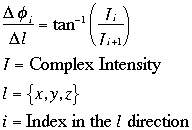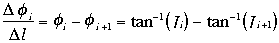Quantitative Measurement of the Phase Error for a Simple and Rapid Phase-Unwrapping Algorithm

G.S. Sobering#+, Y. Shiferaw*, P. van Gelderen*, C.T.W. Moonen*

*In Vivo NMR Research Center
NIH, Bethesda, MD, USA
+Present address: GE Medical Systems, Milwaukee, WI, USA

Poster at 1995 SMR Annual Meeting

Introduction:

There are many applications which require the creation of MR phase images. In our lab, we are particularly interested in obtaining maps of the water frequency to determine static B0 for use as a priori information in spectroscopic imaging analysis and for measuring temperature in vivo via the water chemical shift.

When computing phase maps from complex-valued MR images, the range of phase angles often exceeds ±2radians across the image. This causes artifactual discontinuities (so- called "wraps") in the image where the phase angle jumps from +to -, or -to +. A powerful and simple method for calculating the relative phase between any two points in a complex-valued array (image or 3D volume) is to sum the incremental angular differences between neighboring voxels along a path connecting the two points . The "unwrapping" problem is thus reduced to selecting an algorithm that connects all the voxels in the object to a single reference voxel.

In the ideal case, the phase determined by this unwrapping procedure is path independent. However, in real data, noise and regions of low signal can cause incorrect results. Many algorithms have been developed to address this problem [2-5]. The computational requirements of some of these algorithms are quite steep, especially for 3D data-sets.

In this abstract, we describe the analysis of phase maps generated using a variety of simple path algorithms. These maps demonstrate the insensitivity of this technique to the choice of path selection algorithm for typical MR data. This implies that simple (and rapid) path creation algorithms, using image magnitude to identify "bad" voxels, may be used successfully. Here, one such algorithm is described and evaluated in detail.

Experimental:

Images were collected with a variety of gradient echo pulse sequences on a 1.5T clinical scanner. Data from both phantom and in vivo studies was analyzed. Complex images were computed using standard Fourier reconstruction. Static phase effects were removed by dividing an image acquired at long TE by an otherwise identical short TE image. The spatial derivatives (differences) of the phase maps were computed using the same division process:This method of calculatingØ/l has the advantage that it directly evaluates the difference phase angle between the two locations, even if they happen to be on either side of ±phase-wrap. This means that phase-wraps present in the original image do not affect theØ/l values, unlike the direct method:Two path algorithms were used to generate maps for this analysis. The first is a very simple rectilinear algorithm, which is presented not as a practical method, but to demonstrate the insensitivity of this class of techniques to non-optimal path choice. In this algorithm, the paths follow the imaging grid axes. For example, in a 2D image, one path between (15,10) and (20,25) would move from (15,10) across to (20,10), and then up to (20,25). For 2D images there are two possible paths (denoted: xy and yx) between any two points, and for 3D volumes there are six (xyz, yzx, zxy, yxz, xzy, zyx). In practice, this algorithm has many disadvantages. Most troubling is that many objects cannot be fully unwrapped, because all the rectilinear paths connecting some points inside the object traverse the exterior region. The second path algorithm is more optimal and robust. It is based on a standard seeded region growing algorithm. The algorithm is started with a single-voxel region at the reference point. At every iteration:

1. The list of voxels on the exterior boundry of the region is sorted by magnitude (highest to lowest).
2. For each voxel on the list, the phase of all nearest neighbor voxels that have not already been visited (or been previously marked as below the intensity threshold) is computed using the appropriateØ/l map. The neighbor voxels are marked as visited, and added to the list of boundry voxels for the next iteration.
Iteration continues until the "next iteration boundry voxels" list is empty. This algorithm has a number of advantages:

• It is computationally simple and fast.
• The region will grow around inclusions of "bad" pixels inside the object.
• The selected path is locally optimized in the sense that given an unvisited voxel with multiple visited nearest- neighbors, the path from the highest magnitude neighbor will be used.

Analysis:

In order to evaluate the errors in these methods, phase maps were produced using the two algorithms described above. This gave seven maps produced using very different paths (the six possible rectilinear paths for a 3D volume, and the one from the region-growing method). The differences between the seven phase values were compared by calculating the standard deviation and maximum difference for every point in a large VOI placed inside the object (phantom, brain, etc.). Results for two typical data-sets are shown below. The phantom data was acquired using a two sequential 3D volume FLASH acquisitions with TE1/TE2/TR of 10/30/41ms. SNR in the long-TE image was 60. The in vivo data was acquired from a human brain study using a 3D volume multi-echo FLASH sequence. The first and fourth echoes were used in the analysis, giving a TE1/TE2/TR of 10/40/55ms. SNR in the long-TE image was ca. 100.

Phantom            Brain
--------------     --------------